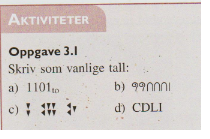User Name Remember Me? Password

 Elementary Math Fractions, Percentages, Word Problems, Equations, Inequations, Factorization, Expansion

 March 13th, 2015, 02:54 PM #1 Member   Joined: Jan 2015 From: Norway Posts: 95 Thanks: 1 Ancient Number SystemsTask 3.1 A)To=two Write as normal numbers A) i have no idea B) 200 + 30 + 1 = 231 C) 2*60^2 + 24*60 + 21 = 7200 + 1440 + 21 = 8621 D) 500 - 100 + 50 + 1 = 400 + 51 = 451March 13th, 2015, 03:07 PM #2 Math Team   Joined: Jul 2011 From: Texas Posts: 3,002 Thanks: 1587 $1101_2 = (1 \times 2^3) + (1 \times 2^2) + (0 \times 2^1) + (1 \times 2^0) = 8 + 4 + 0 + 1 = 13$March 13th, 2015, 03:12 PM   #3
Member

Joined: Jan 2015
From: Norway

Posts: 95
Thanks: 1

Quote:
 Originally Posted by skeeter$1101_2 = (1 \times 2^3) + (1 \times 2^2) + (0 \times 2^1) + (1 \times 2^0) = 8 + 4 + 0 + 1 = 13$
Thanks again i understood itTags ancient, number, systemsThread ToolsShow Printable VersionEmail this Page Display ModesLinear ModeSwitch to Hybrid ModeSwitch to Threaded ModeSimilar Threads Thread Thread Starter Forum Replies Last Post Shen Elementary Math 2 June 5th, 2014 07:50 AM Dawud Math 13 April 20th, 2014 03:41 AM Lars Bo Algebra 1 October 5th, 2013 08:25 AM peterle1 Algebra 2 January 9th, 2010 07:37 AM Lars Bo New Users 0 December 31st, 1969 04:00 PM

 Contact - Home - Forums - Cryptocurrency Forum - Top

Copyright © 2019 My Math Forum. All rights reserved.